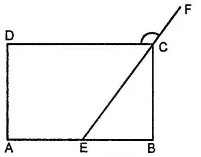Explain how a square is.

(ii) a parallelogram

(iii) a rhombus

(iv) a rectangle

Asked by Aaryan | 1 year ago |  168

##### Solution :-

(i) A square is a quadrilateral since it has four sides.

(ii) A square is a parallelogram since its opposite sides are parallel to each other.

(iii) A square is a rhombus since its four sides are of the same length.

(iv) A square is a rectangle since each interior angle measures 90°.

Answered by Sakshi | 1 year ago

### Related Questions

#### In the given figure, PQRS is a kite. Find the values of x and y.

In the given figure, PQRS is a kite. Find the values of x and y.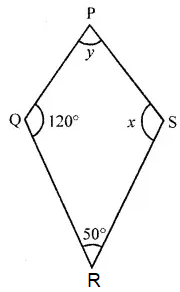#### In the given isosceles trapezium ABCD, ∠C = 102°. Find all the remaining angles of the trapezium.

In the given isosceles trapezium ABCD, ∠C = 102°. Find all the remaining angles of the trapezium.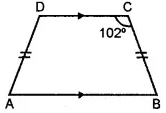#### In the given figure, ABCD is a rhombus and ∠ABD = 50°. Find :

In the given figure, ABCD is a rhombus and ∠ABD = 50°. Find :

(i) ∠CAB

(ii) ∠BCD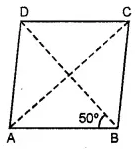#### In the given figure, ABCD is a rectangle and diagonals intersect at O. If ∠AOB = 118°, find

In the given figure, ABCD is a rectangle and diagonals intersect at O. If ∠AOB = 118°, find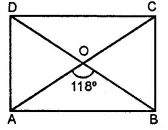(i) ∠ABO

(iii) ∠OCB